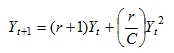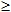### Mark W. Denny, Luke J. H. Hunt, Luke P. Miller, and Christopher D. G. Harley. 2009. On the prediction of extreme ecological events. Ecological Monographs 79:397–421.

Appendix D. Disturbance effects on logistic growth.

The logistic equation provides a simple model of population size, in which the growth of the population through time is governed by both its intrinsic rate of increase r and the carrying capacity of the environment C:(D.1)

Here Yt is the number of individuals in the population at time t and Yt+1 is population size at time t+1.When population size is small, growth is exponential, but growth rate decreases as population size approaches carrying capacity. In the example we use here, r = 0.05, C = 10,000.

To model the disturbance resulting from an extreme event, we reduce population size by an intensity factor E. That is, if population size immediately before the event is Y, size immediately after the event is Y/E. Periodic imposition of disturbance is akin to sustainably harvesting a stock of fish; after an initial period of equilibration, population size fluctuates about a steady average. It is the more complex effect of randomly-imposed events that is of interest here.

In our heuristic example, random extreme events (with a given intensity E) have a probability P = 0.97 of not occurring in a given time interval. In other words, in each interval we pick a random number from a uniform distribution between 0 and 1. If that number is < 0.97, no extreme event is imposed. If the random number0.97, the population size is reduced by a factor of E. The system is initialized with 10 individuals and allowed to run for 500 intervals, allowing the process to “equilibrate.” We then measure the average population size over the next 10,000 intervals. This entire process is repeated 30 times, and the average and standard deviation of these average population sizes is recorded. For comparison, the same experiment is repeated, but extreme events are imposed every 33.3 intervals (equal to the return time of random events). We varied intensity factor from 1 (= no effect) to 6 (only 1/6 of the population survives each extreme event). Higher intensity factors resulted in extinction (population size < 1 individual) at some point in the 10,000 years.

We present the results of this model solely as a heuristic example to demonstrate potential contrasts between random and periodic disturbances. Different effects can be obtained with different values for r, C, and P.

[Back to M079-014]